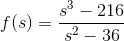# Continuous Extension to a Point (Factoring Question)

## Homework Statement

Define f(6) in a way that extendsto be continuous at s=6.

## Homework Equations

None. Only limits are required.

## The Attempt at a Solution

In order to figure out which point needs to be added to the function, I have to find the limit of this function as s->6. This will result in a zero-denominator, however, so I need to factor [PLAIN]http://latex.codecogs.com/gif.latex?s^2-36 [Broken] [Broken] out of the numerator, so that I can cancel them and use the resulting polynomial to calculate the limit.

Basically, I just need help factoring [PLAIN]http://latex.codecogs.com/gif.latex?s^2-36 [Broken] [Broken] out of [URL]http://latex.codecogs.com/gif.latex?s^3-216;[/URL] I can solve the rest of the question easily.

Help would be appreciated.

Thanks,
Braeden

Last edited by a moderator:

Mark44
Mentor

## Homework Statement

Define f(6) in a way that extendsto be continuous at s=6.

## Homework Equations

None. Only limits are required.

## The Attempt at a Solution

In order to figure out which point needs to be added to the function, I have to find the limit of this function as s->6. This will result in a zero-denominator, however, so I need to factor [PLAIN]http://latex.codecogs.com/gif.latex?s^2-36 [Broken] [Broken] out of the numerator, so that I can cancel them and use the resulting polynomial to calculate the limit.

Basically, I just need help factoring [PLAIN]http://latex.codecogs.com/gif.latex?s^2-36 [Broken] [Broken] out of [URL]http://latex.codecogs.com/gif.latex?s^3-216;[/URL] I can solve the rest of the question easily.
The numerator doesn't have a factor of s2 - 36. There is a linear factor that can be pulled out, however.

Last edited by a moderator:
The numerator doesn't have a factor of s2 - 36. There is a linear factor that can be pulled out, however.

Yeah, I figured it out, thanks. I'm not quite sure what I was trying to do. I ended up factoring (x-6) out of the numerator and denominator and cancelling those.

Mark44
Mentor
Hopefully, you factored out s - 6 rather than x - 6.

Hopefully, you factored out s - 6 rather than x - 6.

Oh yeah.. sorry. I factored (s-6)